Question

# Which of the following statements is correct? The internal energy of a system increases when more...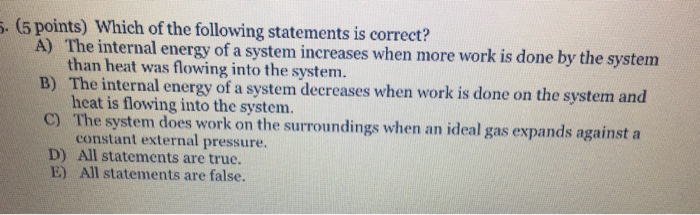Which of the following statements is correct? The internal energy of a system increases when more work is done by the system than heat was flowing into the system. The internal energy of a system decreases when work is done on the system and heat is flowing into the system. The system does work on the surroundings when an ideal gas expands against a constant external pressure. All statements are true. All statements are false.

(A) is correct

(B) is correct

(C) is correct

So all the given statements given are true.

Therefore option (D) is correct

#### Earn Coins

Coins can be redeemed for fabulous gifts.

Similar Homework Help Questions
• ### 1. A gas absorbs 0.0 J of heat and then performs 30.8 J of work. The change in internal energy of the gas is a. 61....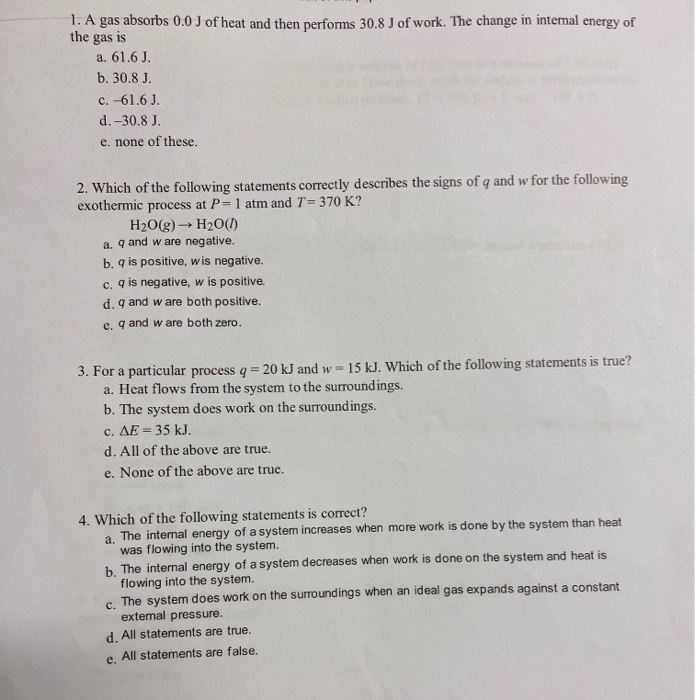1. A gas absorbs 0.0 J of heat and then performs 30.8 J of work. The change in internal energy of the gas is a. 61.6 J. b. 30.8 J. c. -61.6 J. d.-30.8 J. e. none of these 2. Which of the following statements correctly describes the signs of q and w for the following exothermic process at P= 1 atm and T = 370 K? H2O(g) → H2O(1) a. q and w are negative. b. is positive, wis...

• ### Consider each of the following processes and determine if work is done by the system, work...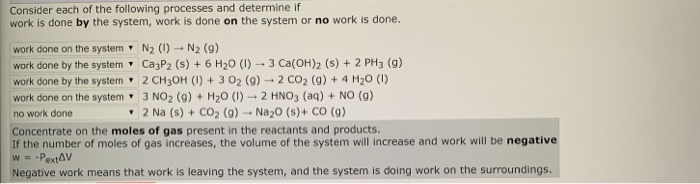Consider each of the following processes and determine if work is done by the system, work is done on the system or no work is done. work done on the system N2 (1) N2 (9) work done by the system Ca3P2 (s) + 6 H20 (1) + 3 Ca(OH)2 (s) + 2 PH3 (9) work done by the system' 2 CH3OH (1) + 3 02 (9) 2 CO2 (9) + 4H20 (1) work done on the system 3 NO2 (9)...

• ### 2. A gaseous system absorbs 43 J of heat and increases its internal energy by 64...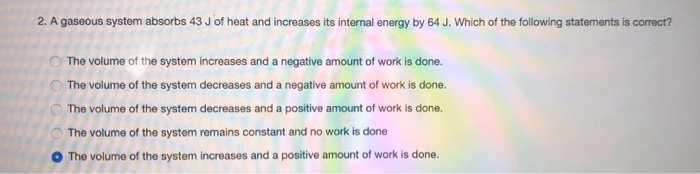2. A gaseous system absorbs 43 J of heat and increases its internal energy by 64 J. Which of the following statements is correct? The volume of the system increases and a negative amount of work is done. The volume of the system decreases and a negative amount of work is done. The volume of the system decreases and a positive amount of work is done. The volume of the system remains constant and no work is done The volume...

• ### Which of these statements are true? -The internal energy of any gas depends only the temperature...

Which of these statements are true? -The internal energy of any gas depends only the temperature of the system. - The internal energy of a gas always increases with temperature -The heat capacity of a monoatomic ideal gas is always smaller than the heat capacity of a polyatomic ideal gas. - q = 0 for any process that does not result in a change in temperature.

• ### Which of the following statements about pressure-volume work is true? The reversible process provides the maximum amou...

Which of the following statements about pressure-volume work is true? The reversible process provides the maximum amount of work. Work done at constant-pressure is zero. When work is done on the system in an adiabatic process there is a decrease in the internal energy of the system. Changes in pressure-volume work must always be offset by changes in the heat of the system. A free expansion takes place against a constant external pressure of 1 bar.

• ### A gaseous system releases 23 J of heat and increases its internal energy by 60 J....

A gaseous system releases 23 J of heat and increases its internal energy by 60 J. Calculate the amount of work associated with this scenario. 83 J of work is done by the system on the surroundings. The system does no work on the surroundings. 37 J of work is done by the system on the surroundings. 83 J of work is done on the system by the surroundings. 37 J of work is done on the system by the...

• ### Part A An ideal gas expands through an adiabatic process. Which of the following statements is/are true? Check all that...

Part A An ideal gas expands through an adiabatic process. Which of the following statements is/are true? Check all that apply. Check all that apply. The work done by the gas is negative, and heat must be added to the system. The work done by the gas is positive, and no heat exchange occurs. The internal energy of the system has increased. The internal energy of the system has decreased. SubmitHintsMy AnswersGive UpReview Part Incorrect; Try Again; 5 attempts remaining...

• ### 24. Suppose that an ideal gas is inside a container with a movable piston. Which of...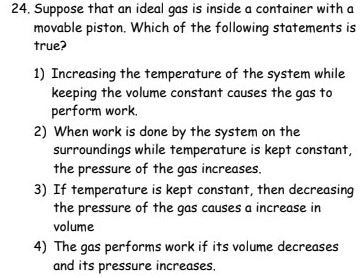24. Suppose that an ideal gas is inside a container with a movable piston. Which of the following statements is true? 1) Increasing the temperature of the system while keeping the volume constant causes the gas to perform work. 2) When work is done by the system on the surroundings while temperature is kept constant, the pressure of the gas increases. 3) If temperature is kept constant, then decreasing the pressure of the gas causes a increase in volume 4)...

• ### Question 11 Ip Which statement is true of the internal energy (AU) of a system and...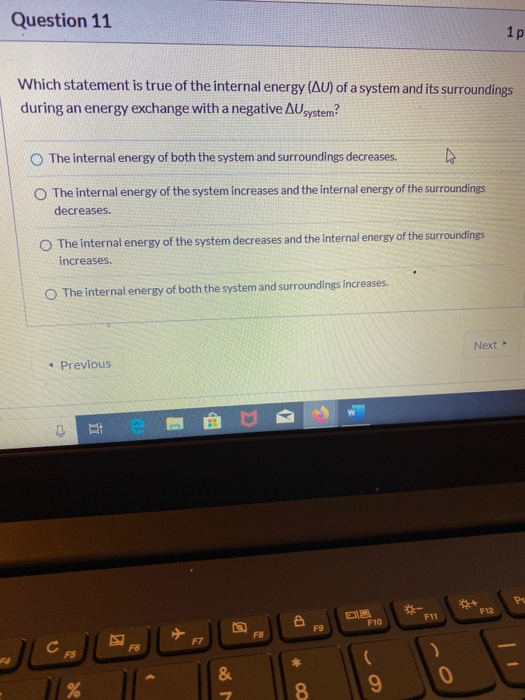Question 11 Ip Which statement is true of the internal energy (AU) of a system and its surroundings during an energy exchange with a negative Ausystem? The internal energy of both the system and surroundings decreases The internal energy of the system increases and the internal energy of the surroundings decreases. The internal energy of the system decreases and the internal energy of the surroundings increases. The internal energy of both the system and surroundings increases. Next → Previous CES...

• ### Describe a process in which the internal energy of some system increases as a result of...

Describe a process in which the internal energy of some system increases as a result of work done on the system by its surroundings. Be sure to identify all objects included in your system.